Function Repository Resource:

# ClosestPrime

Find the prime integer closest to a number

Contributed by: Wolfram|Alpha Math Team
 ResourceFunction["ClosestPrime"][n] returns the prime number closest to n.

## Details and Options

If the two primes nearest to n are equally distant, ResourceFunction["ClosestPrime"][n] returns the lower of the two.

## Examples

### Basic Examples (2)

Find the closest prime to 100:

 In:=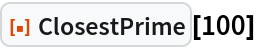Out=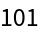Find the closest prime to 15:

 In:=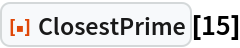Out=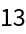### Properties and Relations (3)

The input to ClosestPrime need not be an integer:

 In:=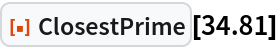Out=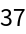Symbolically specified numbers may also be used:

 In:=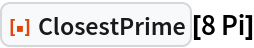Out=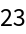Negative numbers are not considered to be prime. Inputting a negative number will always return 2:

 In:=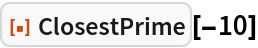Out=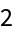ClosestPrime is similar but not identical to NextPrime. ClosestPrime[n] returns the prime number to closest to n, considering numbers less than, equal to, or greater than n. NextPrime[n] returns the closest prime number to n, considering only numbers greater than n:

 In:=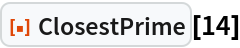Out=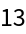In:=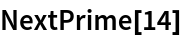Out=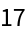## Publisher

Wolfram|Alpha Math Team

## Version History

• 2.0.0 – 23 March 2023
• 1.1.0 – 12 May 2021
• 1.0.0 – 10 April 2020